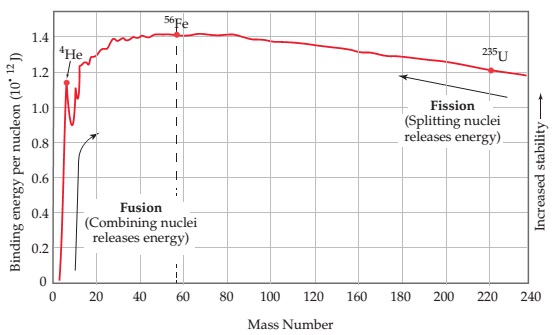# Problem: Which of the following nuclei is likely to have the largest mass defect per nucleon: 11B, 51V, 118Sn, 243Cm?

###### FREE Expert Solution

We’re being asked to determine which of the following nuclei is likely to have the largest mass defect per nucleon: 11B, 51V, 118Sn, 243Cm based on the plot of their binding energy.

To do so, we need to do the following steps,

Step 1: Based on the plot, determine the nucleon with the highest binding energy.
Step 2: Determine the nucleon with the largest mass defect (Δm) in kg, using the equation:

$\overline{){\mathbf{E}}{\mathbf{=}}{\mathbf{∆}}{\mathbf{m}}{{\mathbf{c}}}^{{\mathbf{2}}}}$

E = binding energy in J

Δm = mass defect in kg
c = speed of light = 3.0x108 m/s

Step 1: From the plot, we can see that the binding energy of each nucleon increases:

• from left to right  → as mass numbers increase during fusion###### Problem DetailsWhich of the following nuclei is likely to have the largest mass defect per nucleon: 11B, 51V, 118Sn, 243Cm?